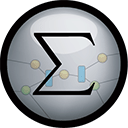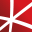# Top 20 NuGet regression Packages

Math.NET Numerics is the numerical foundation of the Math.NET project, aiming to provide methods and algorithms for numerical computations in science, engineering and every day use. Supports .Net Framework 4.0 or higher and .Net Standard 1.3 or higher, on Windows, Linux and Mac.## Math.NET Numerics - Strong Name Edition

Math.NET Numerics is the numerical foundation of the Math.NET project, aiming to provide methods and algorithms for numerical computations in science, engineering and every day use. Supports .Net Framework 4.0 or higher and .Net Standard 1.3 or higher, on Windows, Linux and Mac. This package contain...
F# Modules for Math.NET Numerics, the numerical foundation of the Math.NET project, aiming to provide methods and algorithms for numerical computations in science, engineering and every day use. Supports .Net Framework 4.5 or higher and .Net Standard 1.6 or higher, on Windows, Linux and Mac.## Math.NET Numerics for F# - Strong Name Edition

F# Modules for Math.NET Numerics, the numerical foundation of the Math.NET project, aiming to provide methods and algorithms for numerical computations in science, engineering and every day use. Supports .Net Framework 4.5 or higher and .Net Standard 1.6 or higher, on Windows, Linux and Mac. This pa...
Foundational classes for financial, engineering, and scientific applications, including complex number classes, general vector and matrix classes, structured sparse matrix classes and factorizations, general sparse matrix classes and factorizations, general matrix decompositions, least squares solut...
Classes for data manipulation and statistical computation, including descriptive statistics, probability distributions, combinatorial functions, multiple linear regression, hypothesis testing, analysis of variance, multivariate statistics, partial least squares, and nonnegative matrix factorization.
The GPU-accelerated version of package CenterSpace.NMath. With a few minor exceptions, such as optional GPU configuration settings, the API is identical between CenterSpace.NMath.Premium and CenterSpace.NMath. If using at least .NET Framework 4.6.1 or .NET Core 2.0, we recommend using one of our NMa...## NMath - Standard Library - Windows x64

Foundational classes for financial, engineering, and scientific applications, including complex number classes, general vector and matrix classes, structured sparse matrix classes and factorizations, general sparse matrix classes and factorizations, general matrix decompositions, least squares solut...
Foundational classes for financial, engineering, and scientific applications, including complex number classes, general vector and matrix classes, structured sparse matrix classes and factorizations, general sparse matrix classes and factorizations, general matrix decompositions, least squares solut...
The Extreme Optimization Numerical Libraries for .NET are a set of libraries for numerical computing and data analysis. This is the main package that contains all the core functionality. For optimal performance, we strongly recommend also referencing one of the native packages based on Intel's Mat...
Contains a matrix extension library, along with a suite of numerical matrix decomposition methods, numerical optimization algorithms for constrained and unconstrained problems, special functions and other tools for scientific applications. This package is part of the Accord.NET Framework.
Contains probability distributions, statistical models and methods such as Linear and Logistic regression, Hidden Markov Models, (Hidden) Conditional Random Fields, Principal Component Analysis, Partial Least Squares, Discriminant Analysis, Kernel methods and functions and many other related techniq...
The GPU-accelerated version of package CenterSpace.NMath.Stats. With a few minor exceptions, such as optional GPU configuration settings, the API is identical between CenterSpace.NMath.Stats.Premium and CenterSpace.NMath.Stats.
Extreme Optimization Numerical Libraries for .NET Data Access Library for JSON.## NMath - Standard Library - OSX x64 (alpha)

Foundational classes for financial, engineering, and scientific applications, including complex number classes, general vector and matrix classes, structured sparse matrix classes and factorizations, general sparse matrix classes and factorizations, general matrix decompositions, least squares solut...
The Extreme Optimization Numerical Libraries for .NET are a set of libraries for numerical computing and data analysis. This package provides types specialized to single-precision, including complex numbers, as well as optimized implementations of single-precision linear algebra. Supports .NET Fra...
The Extreme Optimization Numerical Libraries for .NET are a set of libraries for numerical computing and data analysis. This package provides a complete implementation of linear algebra functionality for arbitrary numerical types. Supports .NET Framework 3.5, 4.0 and 4.6+, .NET Standard 1.3 and 2...
Provides learning algorithms and models for DecisionTree regression and classification.
Provides learning algorithms and models for RandomForest and ExtraTrees regression and classification.
Provides ensemble learning for regression and classification.